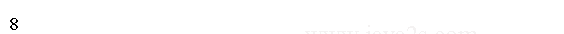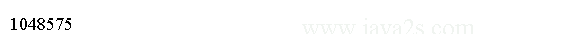# Java int type

In this chapter you will learn:

### Description

When byte and short values are used in an expression they are promoted to int when the expression is evaluated.

### Size and value

`int` is a signed 32-bit type that has a range from `-2,147,483,648` to `2,147,483,647`.

### octal integer(base eight)

Octal values are denoted in Java by a leading zero. valid value 09 will produce an error from the compiler, since 9 is outside of octal's 0 to 7 range.

``````
public class Main {
/*from  w w w  .ja  v a 2  s .  c o m*/
public static void main(String[] args) {
int i = 010;

System.out.println(i);
}
}
``````

The output:### hexadecimal integer(base 16)

hexadecimal matches with modulo 8 word sizes, such as 8, 16, 32, and 64 bits. You signify a hexadecimal constant with a leading zero-x, (0x or 0X).

The range of a hexadecimal digit is 0 to 15, so A through F (or a through f ) are substituted for 10 through 15.

An integer literal can always be assigned to a long variable. An integer can also be assigned to a char as long as it is within range.

``````
public class Main{
public static void main(String[] argv){
int f = 0XFFFFF;
//from  w  ww  .ja v a2s  .co  m
System.out.println(f);//1048575

}
}
``````

The code above generates the following result.#### Next chapter...

What you will learn in the next chapter:

Home »
Java Tutorial »
Java Langauge »
Java Data Types
Java Primitive Data Types
Java boolean type
Java char type
Java char value escape
Java byte type
Java short type
##### Java int type
Java long type
Java float type
Java double type
Java String type
Java String Escape
Java String Concatenation